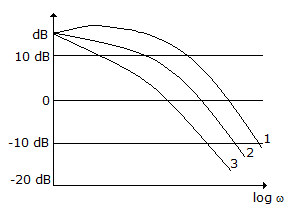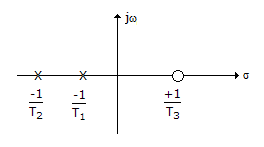# Electronics and Communication Engineering - Automatic Control Systems

### Exercise :: Automatic Control Systems - Section 6

16.

The given figure shows log magnitude versus ω plots of a system for different values of gain. For this systemA. curve 1 shows highest gain margin and curve 2 shows poorest gain margin B. gain margins shown by the three curves are equal C. curve 1 shows poorest gain margin and curve 3 shows highest gain margin D. curve 3 shows poorest gain margin while curve 1 shows highest gain margin

Explanation:

No answer description available for this question. Let us discuss.

17.

The purpose of series quadrature windings in amplidyne is to

 A. neutralize the effect of armature reaction B. reduce commutation difficulties C. increase the gain D. increase response time

Explanation:

No answer description available for this question. Let us discuss.

18.

For the transport lag G(jω) = e-jωT, the phase angle

 A. is constant B. varies linearly with frequency C. varies linearly with low frequencies only D. values as per square law

Explanation:

No answer description available for this question. Let us discuss.

19.

In classical analogue, torque is analogous to

 A. current B. voltage C. charge D. voltage gradient

Explanation:

No answer description available for this question. Let us discuss.

20.

The pole zero plot of the given figure is forA. non-minimum phase function B. minimum phase function C. all pass function D. either (b) or (c)